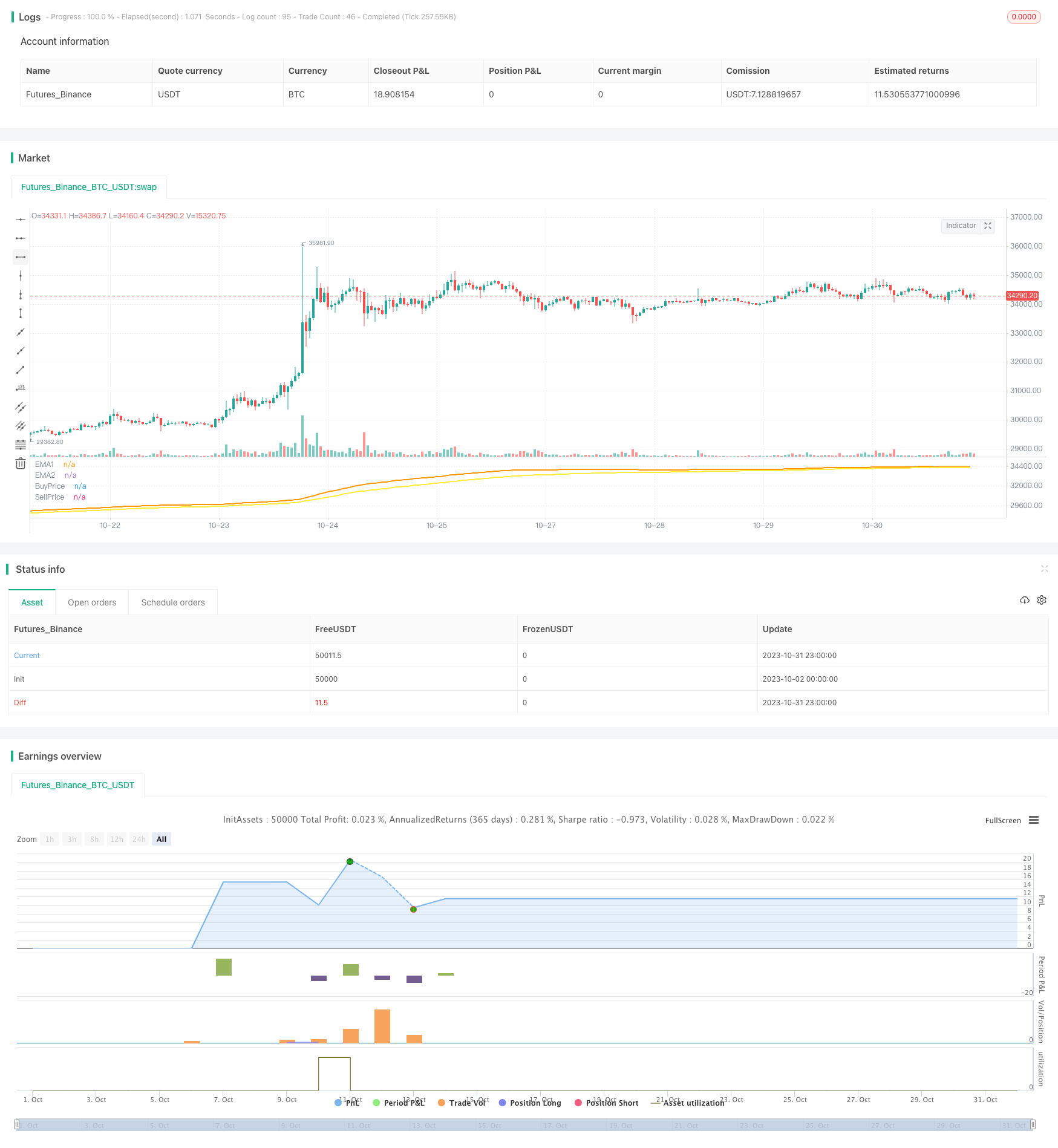# Martingale Strategy with Expanded Moving Average Range for Stock Trading

Author: ChaoZhang, Date: 2023-11-02 17:16:08
Tags:### Overview

This strategy identifies trends by expanding the interval between moving averages. When an upward trend is identified, it gradually builds up long positions to profit from the trend. At the same time, stop loss points are set to control risks.

### Strategy Logic

1. Set two moving averages, EMA1 and EMA2, with slightly different periods, e.g. 55 and 89. This creates a wider range between the MAs.

2. When price breaks above the MAs, it signals an upward trend. Long positions can then be gradually built up.

3. After taking a position, continue pyramiding when price keeps rising. This allows higher profits from the trend.

4. Set a stop loss point below the MAs. When price drops below the MA, close longs to stop loss. The stop loss floats up with entry price.

5. This allows pyramiding positions to profit from a trend, while setting a stop loss to control risks.

1. The wider MA range helps clearly identify trends.

2. Pyramiding creates higher returns from trends.

3. Dynamic stop loss takes profits from trends while limiting losses.

4. Suitable for long-term trend trading.

### Risk Analysis

1. Trend must be correctly identified, otherwise losses accelerate.

2. Pyramiding must be controlled to avoid margin call risks.

3. Stop loss must be reasonably set, too wide may expand losses, too tight may cause whipsaws.

4. Liquidity must be considered, low liquidity assets are unsuitable.

### Optimization Suggestions

1. Add more indicators like RSI, KD to confirm trends and avoid false breakouts.

2. Optimize MA periods based on asset characteristics to find best combinations.

3. Research optimal pyramiding models to control position sizing risks.

4. Consider partial profit taking to lock in gains and reduce drawdowns.

5. Set stop loss based on asset volatility to balance protection and avoiding whipsaws.

### Summary

This strategy identifies trends with wider MA range, pyramids positions to profit from trends, and sets a floating stop loss to control risks. It can achieve good returns in trends, but needs further optimization on entry signals, pyramiding, stop loss etc. to become more robust across different assets.

```/*backtest
start: 2023-10-02 00:00:00
end: 2023-11-01 00:00:00
period: 1h
basePeriod: 15m
exchanges: [{"eid":"Futures_Binance","currency":"BTC_USDT"}]
*/

//@version=2
// strategy(title='Super Simple Martingale Buying', shorttitle="Martingale v5",overlay=true, pyramiding = 10, initial_capital=1, calc_on_order_fills = true)

// Revision:        1
// Author:          @ToS_MavericK

// === INPUT SMA ===
EMA1  = input(55)
EMA2  = input(89)

Amount  = input(defval = 6, type = float, title = "Max open Orders", minval = 1, step = 1)
Multiplier  = input(defval = 2  , type = float, title = "Multiplier", minval = 1, step = 0.1)
BuyLvl  = input(defval = 1, type = float, title = "BuyLvl", minval = 0, step = 0.1)
Profit  = input(3)
DoubleUpLimit    = input(2)

// === INPUT BACKTEST RANGE ===
FromDay = input(defval = 1, title = "From Day", minval = 1, maxval = 31)
FromMonth   = input(defval = 1, title = "From Month", minval = 1, maxval = 12)
FromYear    = input(defval = 2019, title = "From Year", minval = 2012)
ToDay   = input(defval = 1, title = "To Day", minval = 1, maxval = 31)
ToMonth = input(defval = 1, title = "To Month", minval = 1, maxval = 12)
ToYear  = input(defval = 2020, title = "To Year", minval = 2012)

RSIFilter = input(false)
minRSI  = input(defval = 35,  title = "RSI", minval = 1, step = 1)
lengthRSI = input(14, minval=1)
src = input(close, title="RSI Source")

StochRSIFilter = input(false)
lengthStoch = input(14, minval=1)
smoothK = input(3, minval=1)
smoothD = input(3, minval=1)

rsi = rsi(src, lengthRSI)
k = sma(stoch(rsi, rsi, rsi, lengthStoch), smoothK)
d = sma(k, smoothD)

// === FUNCTION EXAMPLE ===
start     = timestamp(FromYear, FromMonth, FromDay, 00, 00)  // backtest start window
finish    = timestamp(ToYear, ToMonth, ToDay, 23, 59)        // backtest finish window
window()  => true // create function "within window of time"

// === SERIES SETUP ===
vEMA1 = ema(close, EMA1)
vEMA2 = ema(close, EMA2)

buy  =  (rsi < minRSI or RSIFilter == false) and ((crossover(k,d) and k < 20) or StochRSIFilter == false) and ((close < vEMA1 * (1 - BuyLvl/100) and vEMA1 < vEMA2) or (close < vEMA2 * (1 - BuyLvl/100) and vEMA2 < vEMA1))

BuyPrice = strategy.position_avg_price * (1 - DoubleUpLimit/50)
SellPrice = strategy.position_avg_price * (1 + Profit/(100*strategy.opentrades))

// Exit first, due to the limit orders, which can be hit on the same bar
strategy.exit("EMA1", limit = SellPrice, when = window() and strategy.opentrades > 0)
strategy.close("EMA1",when = time > finish) // close positions at the end of the specified time period

// Normal entry
strategy.entry("EMA1", strategy.long,qty = strategy.equity/ (close * pow(2,Amount - 1)), when = window() and strategy.opentrades == 0 and buy)
// Martingale
strategy.entry("EMA1", strategy.long,qty = strategy.position_size, limit = strategy.position_avg_price * (1 - DoubleUpLimit/100), when = window() and strategy.opentrades == 1)
strategy.entry("EMA1", strategy.long,qty = strategy.position_size, limit = BuyPrice, when = window() and strategy.opentrades > 1 and strategy.opentrades < Amount)

plot(vEMA1, title = 'EMA1', color = orange, linewidth = 2, style = line)
plot(vEMA2, title = 'EMA2', color = yellow, linewidth = 2, style = line)
plot(BuyPrice, title = 'BuyPrice', color = red, linewidth = 2, style = line)
plot(SellPrice, title = 'SellPrice', color = green, linewidth = 2, style = line)
```

More